# ML 105: Classification (15 pts extra)

## What You Need

• A Web browser

## Purpose

To practice making simple machine learning code in Python. This project was adapted from chapter 2 of this book:

In a browser, go to

From the menu, click File, "New notebook".

# Getting the Data

Execute these commands to download the MNIST dataset, which contains 70,000 small images of handwritten digits.
```from sklearn.datasets import fetch_openml mnist = fetch_openml('mnist_784', as_frame=False, parser="auto") print(mnist.DESCR) ```
As shown below, a description of the data appears.## Understanding the Data

Execute these commands to put the data in variables X and y and display it in various ways:
```X, y = mnist.data, mnist.target print(X) print(X.shape) print(y) print(y.shape) ```
As shown below, X contains 70000 records, each with 784 values. These are the pixels of a 28x28 image.

y contains the number that was written, for all 70000 images. Notice that the first two images are '5'and '0'.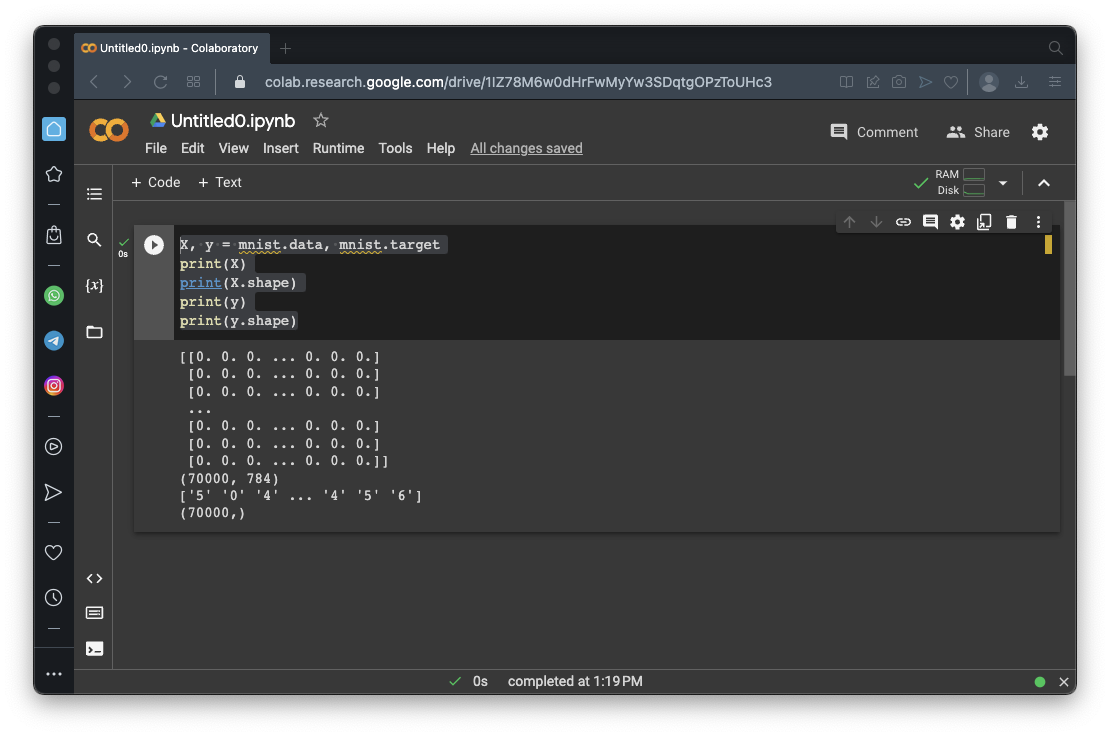## Viewing the Images

Execute these commands to see the first 100 images:
```import matplotlib.pyplot as plt def plot_digit(image_data): image = image_data.reshape(28, 28) plt.imshow(image, cmap="binary") plt.axis("off") plt.figure(figsize=(9, 9)) for idx, image_data in enumerate(X[:100]): plt.subplot(10, 10, idx + 1) plot_digit(image_data) plt.subplots_adjust(wspace=0, hspace=0) plt.show() ```
As shown below, the images are mostly recognizable, but vary a lot.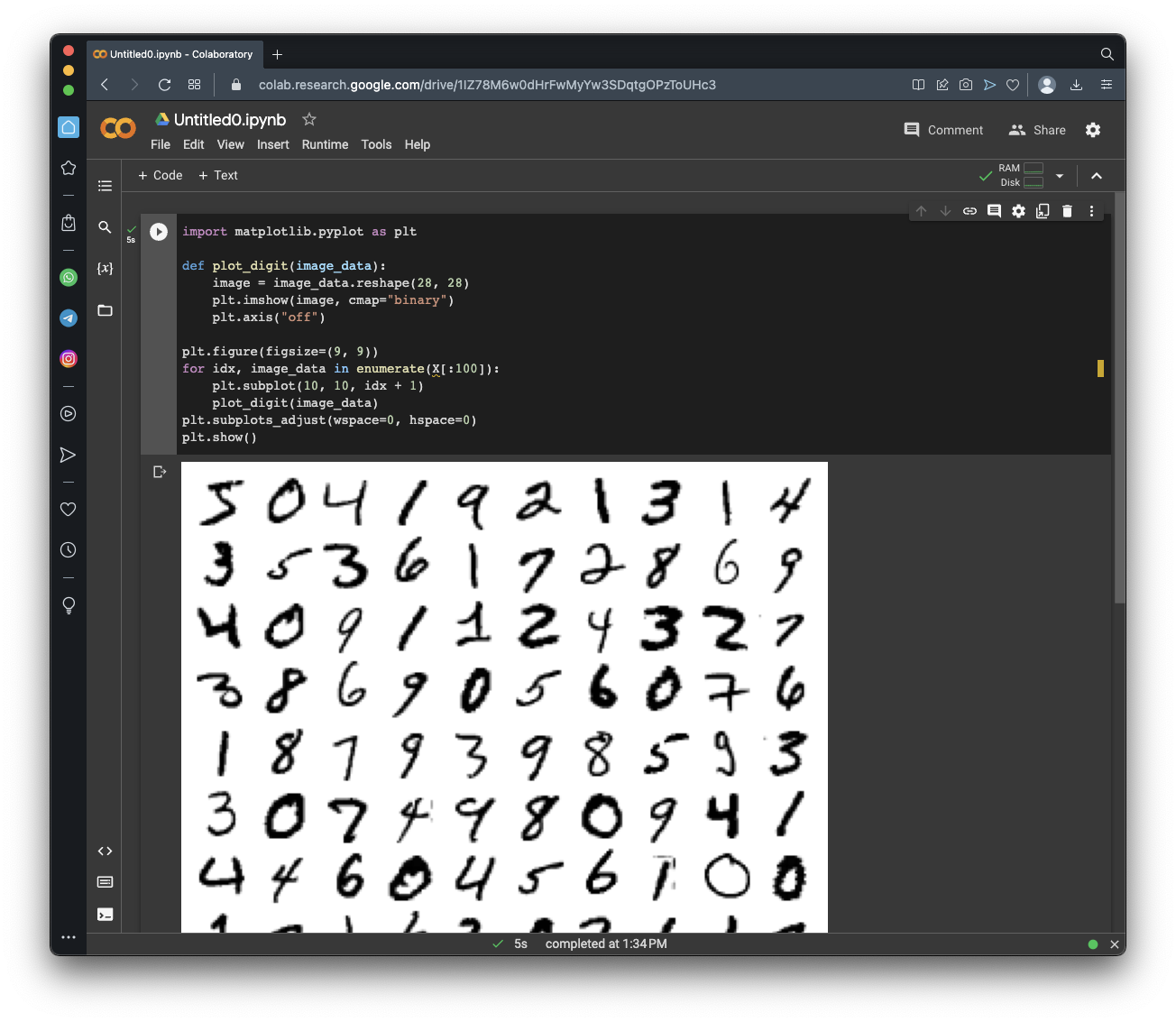## Training and Testing Sets

Execute these commands to create training and testing sets:
```X_train, X_test, y_train, y_test = X[:60000], X[60000:], y[:60000], y[60000:] print("Training set:", len(X_train)) print("Test set:", len(X_test)) ```
As shown below, the training set contains 60,000 images and the training set contains 10,000 images.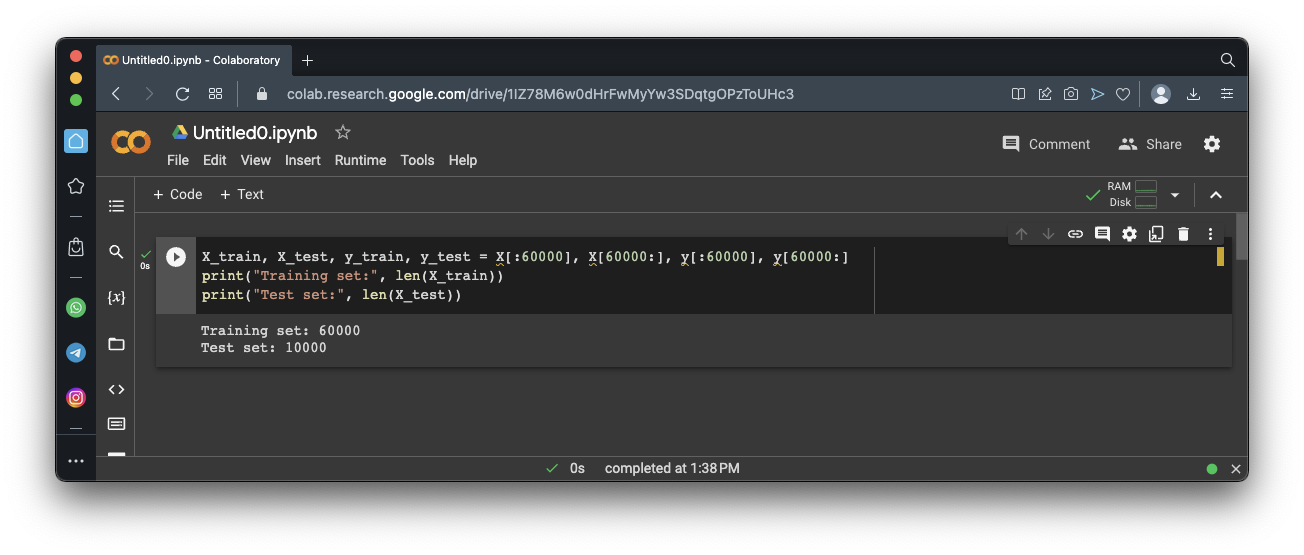## Praparing Binary Data

Execute these commands to create a simple dataset marking all the '5' images as 'True', and the others 'False':
```import numpy y_train_5 = (y_train == '5') # True for all 5s, False for all other digits y_test_5 = (y_test == '5') print("Training set:", y_train_5) print("True:", numpy.count_nonzero(y_train_5 == True)) print("False:", numpy.count_nonzero(y_train_5 == False)) print() print("Test set:", y_test_5) print("True:", numpy.count_nonzero(y_test_5 == True)) print("False:", numpy.count_nonzero(y_test_5 == False)) ```
As shown below, both sets are mostly 'False'.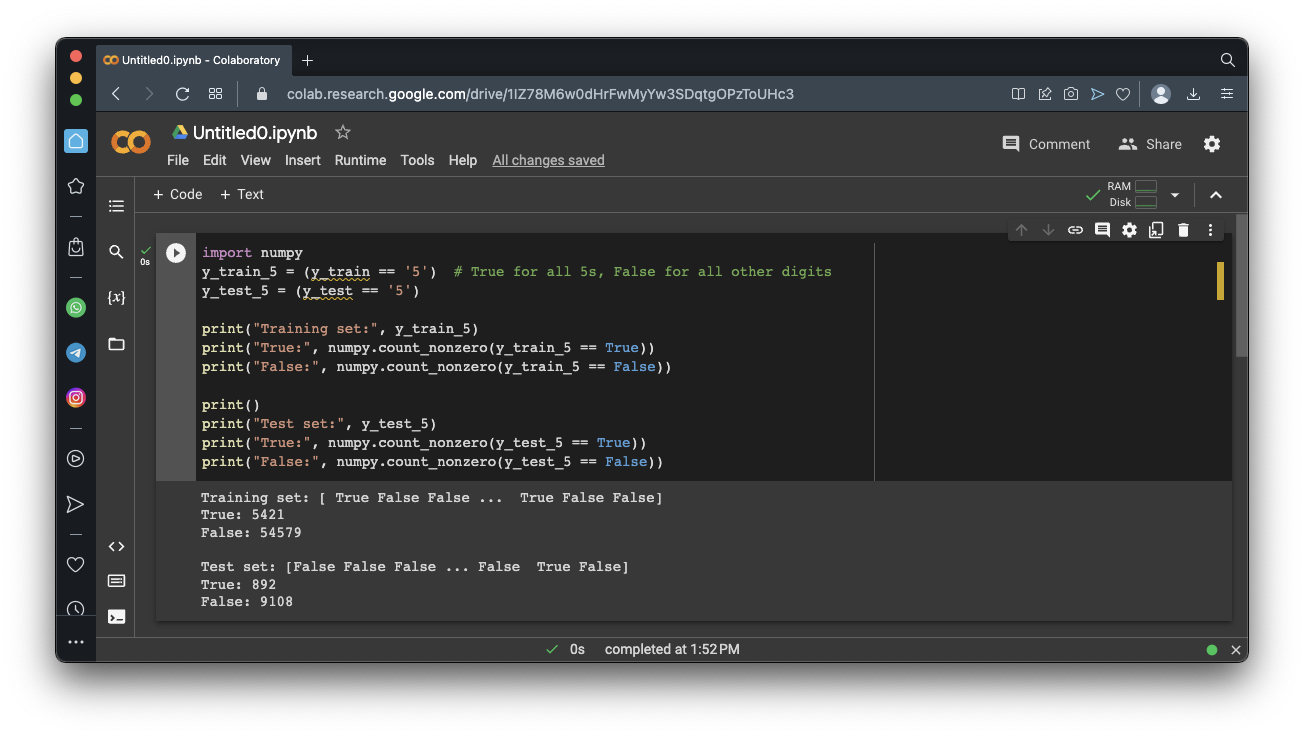## Linear Model

We'll use a linear model -- each pixel is just multiplied by a weight factor and added together to create a single output score, which is used to determine 'True' or 'False' predictions.

The learning is a process of choosing the weights.## Training a Binary Classifier

Execute these commands to create and train a "stochastic gradient descent" (SGD) model:
```from sklearn.linear_model import SGDClassifier sgd_clf = SGDClassifier(random_state=42, verbose=2) sgd_clf.fit(X_train, y_train_5) print("Prediction for image 0 (",y,"):", sgd_clf.predict([X])) print("Prediction for image 1 (",y,"):", sgd_clf.predict([X])) print("Prediction for image 2 (",y,"):", sgd_clf.predict([X])) ```
As shown below, the model trains for 239 epochs, and correctly predicts the first three image values (the first one is '5' and the others are not).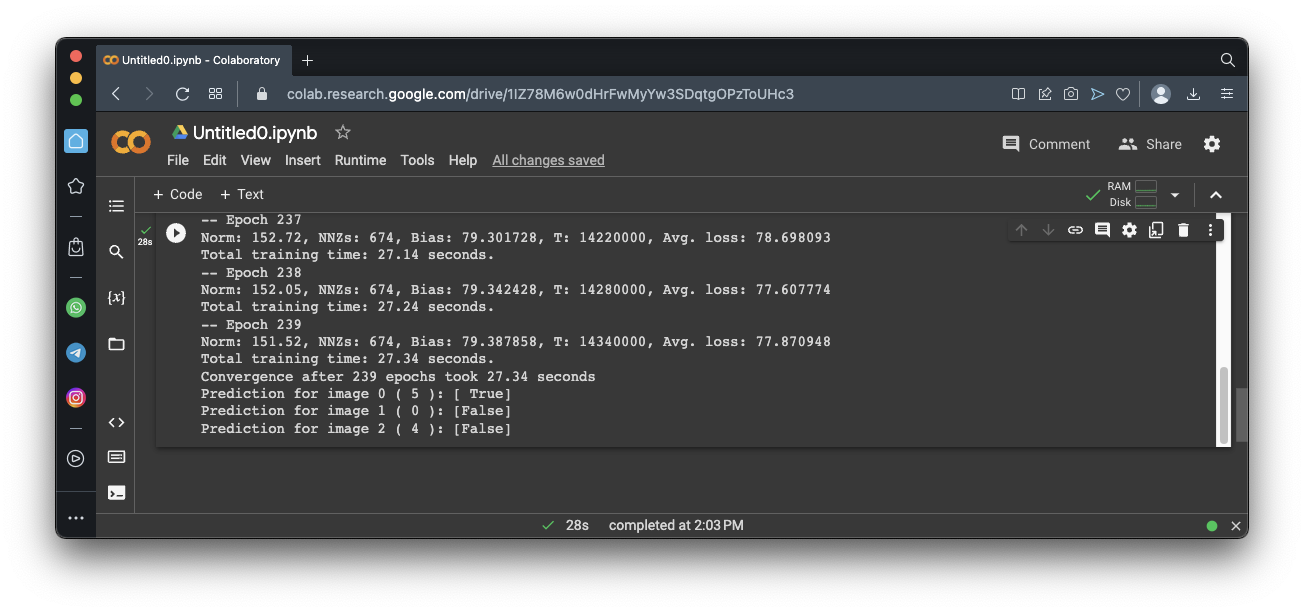## Measuring Accuracy Using Cross-Validation

Cross-validation tests a model by doing these things:
• Split the training data into three "folds"
• Train the model three times, excluding one of the "folds" each time
• Evaluate the accuracy three times using the excluded "folds"
Of course, you could use some number of folds other than three.

Execute these commands to perform cross-validation on our model:

```from sklearn.model_selection import cross_val_score cross_val_score(sgd_clf, X_train, y_train_5, cv=3, scoring="accuracy") ```
As shown below, the three accuracy measures were 95%, 96%, and 96%.

However, remember that our data set is 90% 'False' values, so that is not as good as it sounds.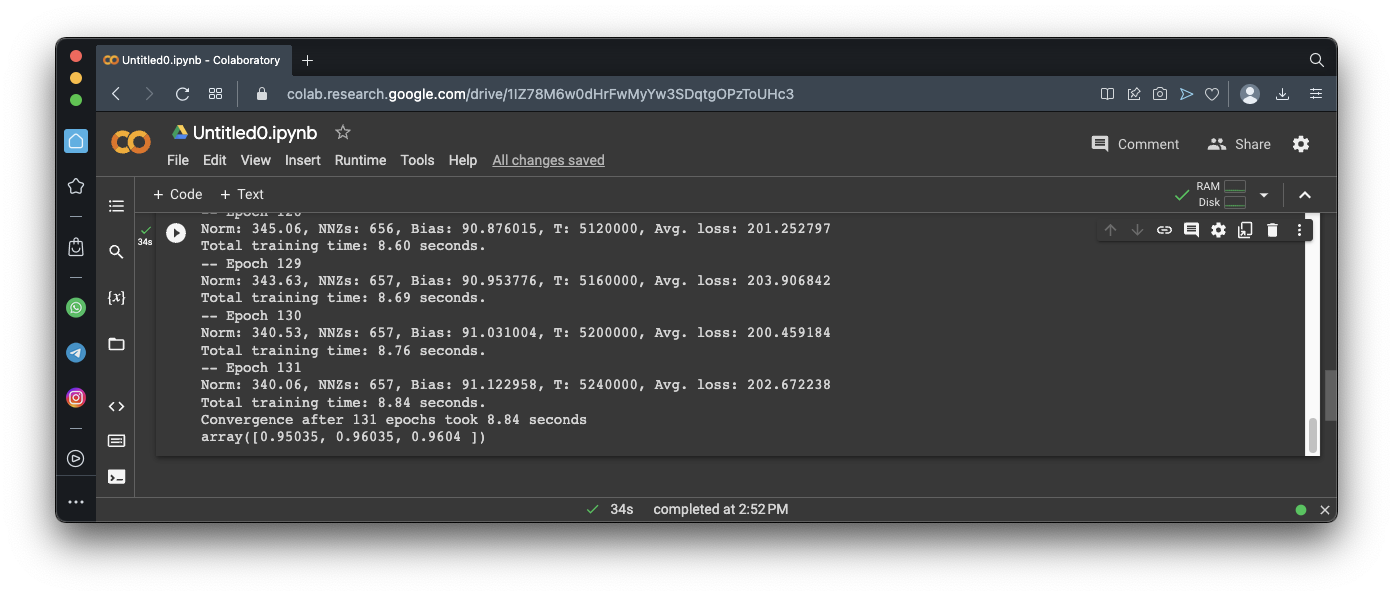## Confusion Matrices

Execute these commands to calculate the "confusion matrix" -- that is, the actual number of wrong predictions from the model.

The cross_val_predict() function will perform 3-fold cross validation, and return the predicted values.

The confusion_matrix() function counts the hits and misses.

```from sklearn.model_selection import cross_val_predict y_train_pred = cross_val_predict(sgd_clf, X_train, y_train_5, cv=3) from sklearn.metrics import confusion_matrix cm = confusion_matrix(y_train_5, y_train_pred) cm ```
The results are in an array, outlined in the image below.

The first row contains most of the images--these are the non-5 images. The model incorrectly called 687 of them 5's.

The second row is the fives. The model incorrectly called 1891 of them non-5.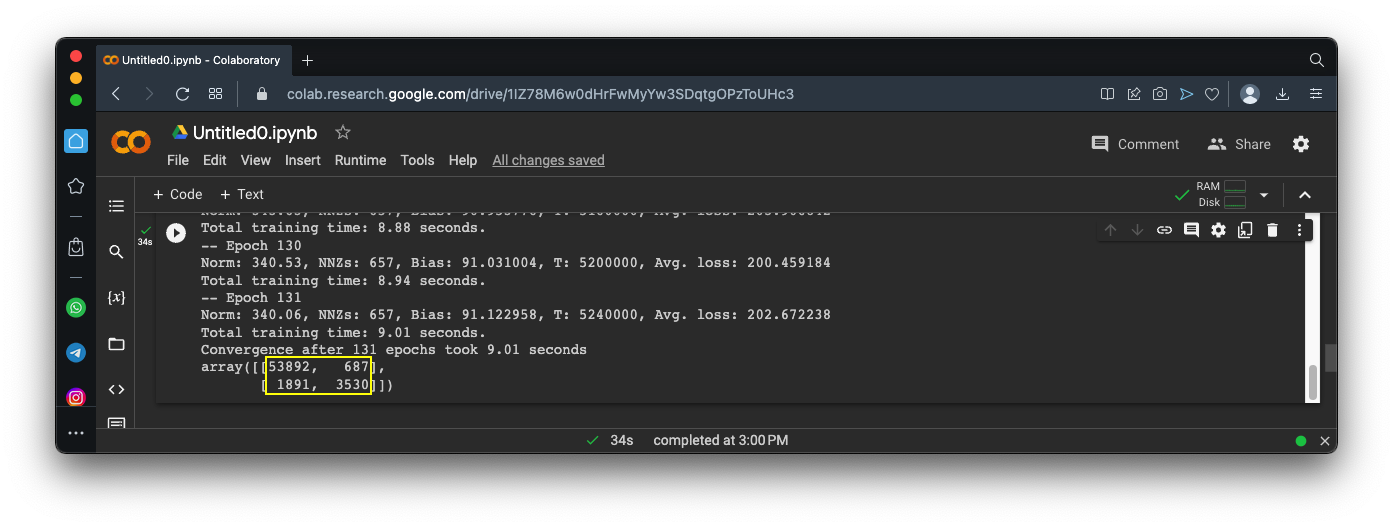## Precision

Precision measures the accuracy of the positive predictions:
Precision = (True Positives) / (True Positives + False Positives)
From the matrix in the image above:
• 687 non-5's were identified as 5's -- these are False Positives
• 3530 5's were identified as 5's -- these are True Positives
So the Precision is
Precision = 3530 / (3530 + 687) = 0.837 = 83.7%
Precision alone is not a good measure of quality, because we could make a model that is very picky, only identifying perfect matches as positive.

This model would have no False Positives, and therefore a Precision of 100%, but it would have many False Negatives.

So a second measure is needed: Recall.

## Recall

Recall measures the precentage of positive instances that were correctly predicted. Recall is also called sensitivity or true positive rate.

Here's the formula for Recall:

Recall = (True Positives) / (True Positives + False Negatives)
From the matrix in the image above:
• 1891 5's were identified as non-5's -- these are False Negatives
• 3530 5's were identified as 5's -- these are True Positives
So the Recall is
Recall = 3530 / (3530 + 1891) = 0.651 = 65.1%

## F1 Score

The F1 score combines precision and recall into a single number called the harmonic mean:
F1 = 2 x (Precision x Recall) / (Precision + Recall)
The F1 score will only be near 1 if both Precision and Recall are. From the values above:
F1 = 2 x (0.837 x 0.651) / (0.837 + 0.651) = 0.732 = 73.2%

## Calculating Precision, Recall, and F1 with sklearn

Execute these commands to calculate the Precision and Recall of your model:
```from sklearn.metrics import precision_score, recall_score, f1_score print("Precision:", precision_score(y_train_5, y_train_pred)) print("Recall:", recall_score(y_train_5, y_train_pred)) print("F1 Score:", f1_score(y_train_5, y_train_pred)) ```
As shown below, the values match the ones calculated above.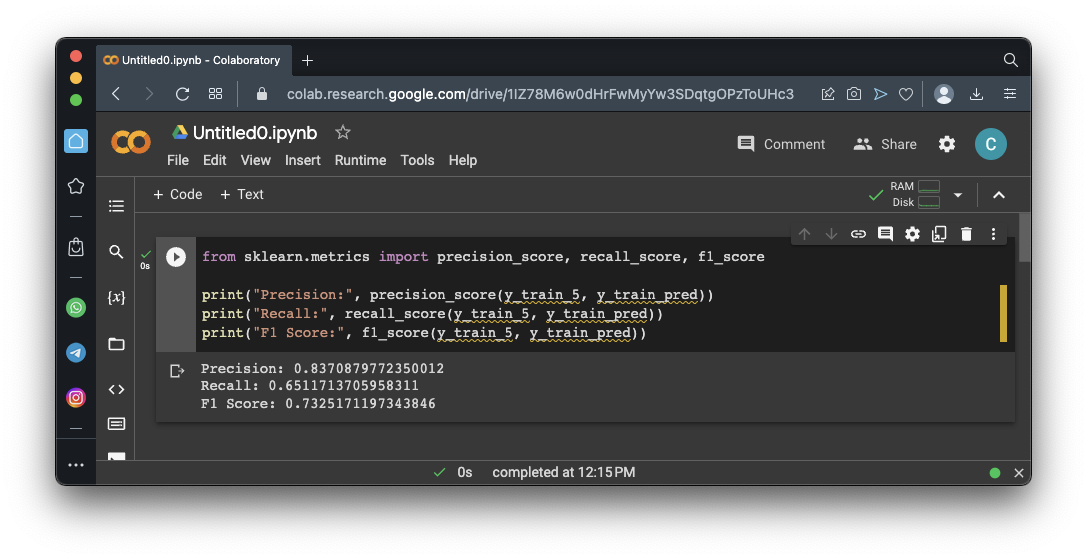As we saw above, by default, sklearn finds a model with approximately equal precision and recall.

But suppose you want asymmetric results--consider a facial recognition scanner to detect known thieves in a retail store. You really want to avoid False Positives, which accuse innocent shoppers of being thieves. It's worth accepting a higher False Negative rate to achieve that end.

Consider the situation shown below.

The neuron has an output signal level. Values above the threshold are classified as 5's, values below that level are classified as non-5's.

If the threshold is low, many outputs are classified as 5's, producing many False Positives. This makes Precision low.

If the threshold is high, many outputs are classified as non-5's, producing many False Negatives. This makes Recall low.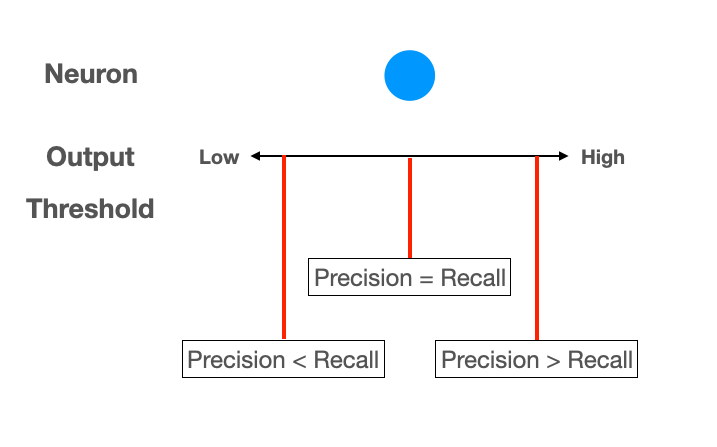## Viewing the Neuron Output

Execute these commands to see the neuron output signal from our model for the first twelve images:
```for i in range(12): print(y[i], sgd_clf.decision_function([X[i]])) ```
As shown below, the signal is only above zero for the first and last images, which are the 5's.

By default, sklearn uses a threshold of zero.

If the threshold were 3000, the first image would be classified as non-5, but the last one would still be classified as 5.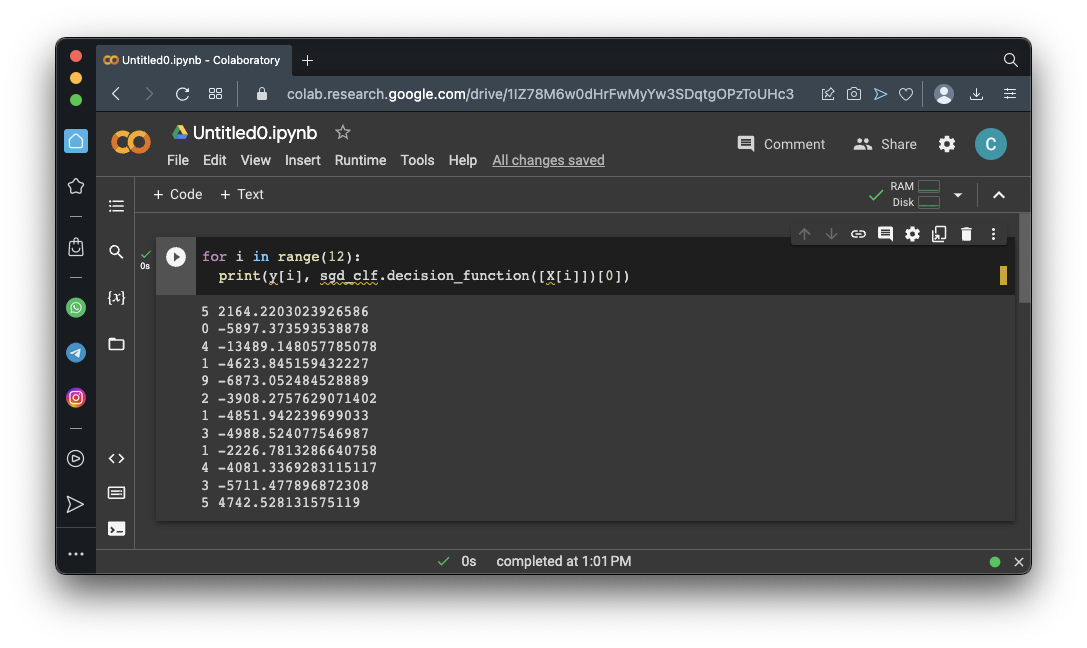## Using Different Threshold Values

Execute these commands to :
```y_scores = cross_val_predict(sgd_clf, X_train, y_train_5, cv=3, method="decision_function") from sklearn.metrics import precision_recall_curve precisions, recalls, thresholds = precision_recall_curve(y_train_5, y_scores) import matplotlib.pyplot as plt plt.plot(thresholds, precisions[:-1], "b--", label="Precision", linewidth=2) plt.plot(thresholds, recalls[:-1], "g-", label="Recall", linewidth=2) plt.grid() plt.axis([-50000, 50000, 0, 1]) plt.xlabel("Threshold") plt.legend(loc="center right") plt.show() ```
As shown below, as the threshold increases, Precision increases and Recall decreases.

Precision gets noisy at high thresholds, where there are very few positives.## Flag ML 105.1: Precision for Threshold 3000 (15 pts)

Execute these commands to calculate the Precision and Recall for a threshold of 0:
```y_train_pred = (y_scores >= 0) print("Precision:", precision_score(y_train_5, y_train_pred)) print("Recall:", recall_score(y_train_5, y_train_pred)) print("F1 Score:", f1_score(y_train_5, y_train_pred)) ```
As shown below, you get the same values we saw above, because the default threshold is zero.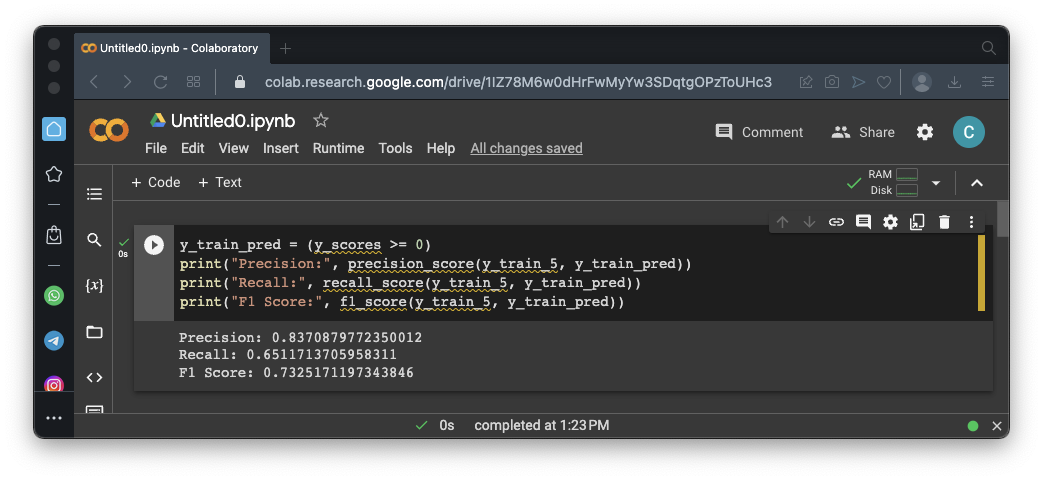Change the zero in the first line to 3000 and calculate the values again. The flag is the precision value, covered by a green rectangle in the image below.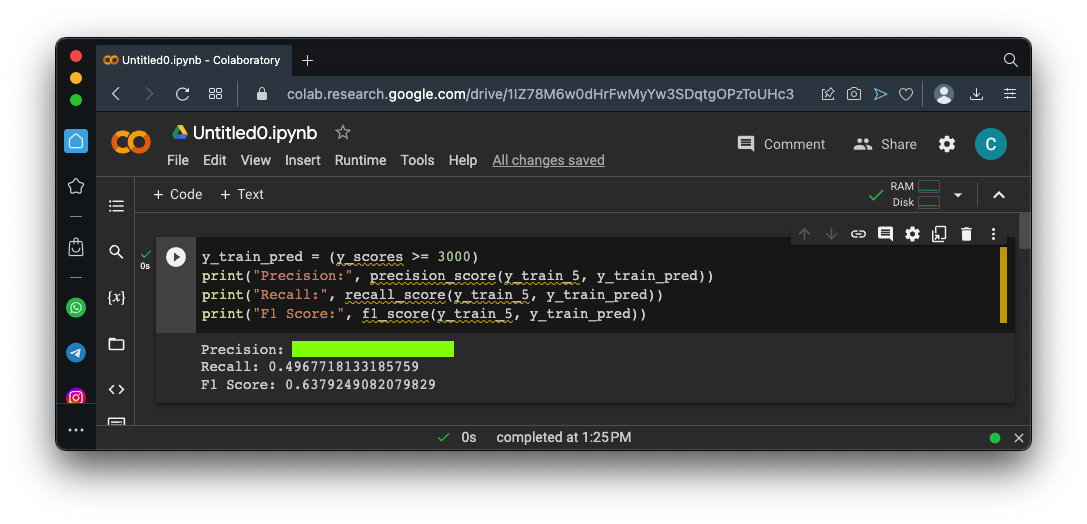## Multiclass Classification

Execute these commands to create and train a model to classify the images into all ten categores, not just "5" and "non-5":
```from sklearn.svm import SVC svm_clf = SVC(random_state=42) svm_clf.fit(X_train[:2000], y_train[:2000]) # y_train, not y_train_5 for i in range(12): print(y[i], svm_clf.predict([X[i]])) ```
The first 12 classifications are shown.

The first column is the right answer, and the second column is the classification from the model.

As shown below, it classified the first 12 images correctly.## Sources

Hands-On Machine Learning with Scikit-Learn, Keras, and TensorFlow 3rd Edition, Kindle Edition